# Setup for 5 Player a Game of Food Chain Magnate

Originally generated on 1/10/2019 11:46:48 PM

Like BoardGameHelpers.com? Then buy the microbadge
on BGG! If I see it displayed under your avatar, I'll refund you the 8gg!

Login to save this setup to My Games

## Player Setup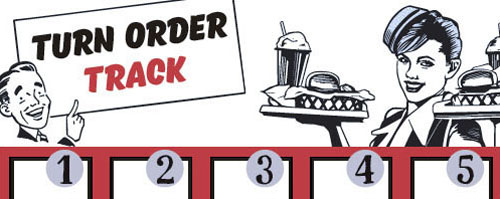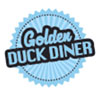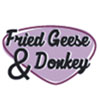## Other Setup

Bank starts with \$250.00
Number of 1x Employee cards used = 3

## Map (Random)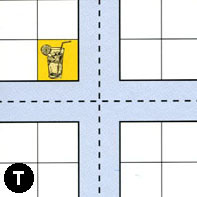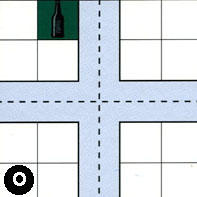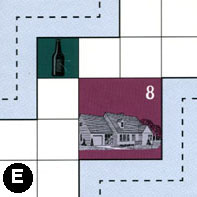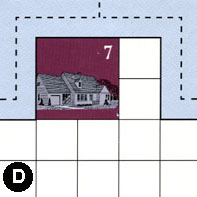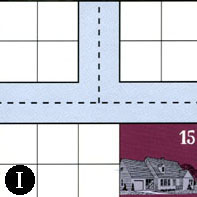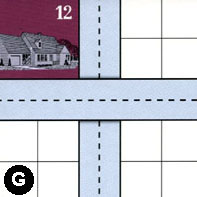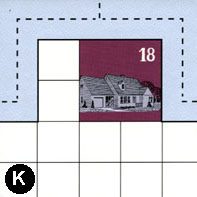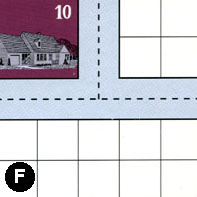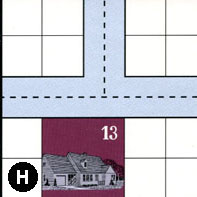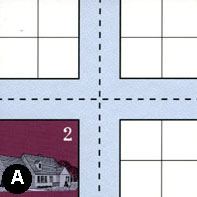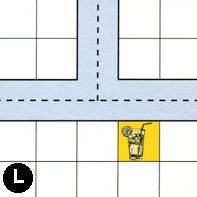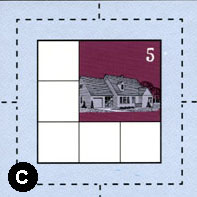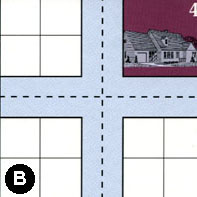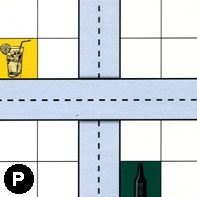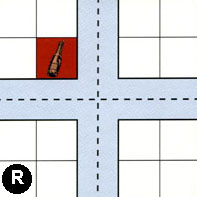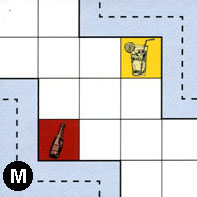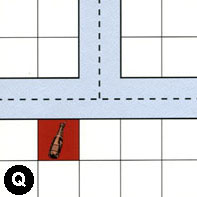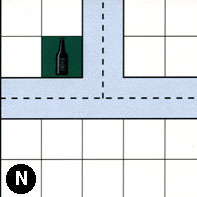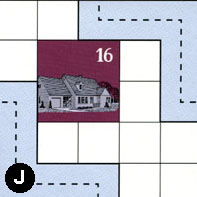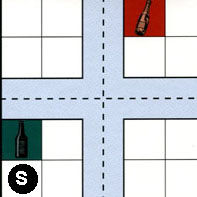### Map Stats

Map Option = Random
Total Number of Tiles = 20
Total Number of Starting Houses = 11
Total Number of Beer Spots = 5
Total Number of Soda Spots = 4
Total Number of Lemonade Spots = 4
Total Number of Drink Spots = 13
Number of independent path systems = 3
Number of independent neighborhoods = 18

Path System 1
Size = Large
Number of Tiles = 20
Number of Paths = 74
Contains Loop(s) = No
Number of Starting Houses = 12
Starting Houses Pct of Map (%) = 109
Number of Beer Spots = 5
Number of Soda Spots = 4
Number of Lemonade Spots = 3
Number of Drink Spots = 12
Drink Spots Pct of Map (%) = 92
Tile Ids = A, B, C, D, E, F, G, H, I, J, K, L, M, N, O, P, Q, R, S, T
Paths = TWE, GNS, LWN, CWE, BWS, BWN, PNS, RWS, AWE, IWN, INE, IWE, AWN, ANE, RWN, RNE, SWN, JEN, PWE, HWE, DWN, EWN, DNE, DWE, HWN, AWS, ANS, AES, RWE, SNE, SWE, SWS, SNS, RES, RNS, SES, HNE, BNE, BWE, BES, CWN, CNE, LNE, MWS, QWE, NWE, JWS, NWN, NNE, QWN, QNE, LWE, CES, KWE, OWS, TWS, TWN, TNE, TES, OWN, ONE, EES, FWN, KWN, ONS, OES, OWE, TNS, KNE, CWS, BNS, CNS, FWE, FNE What do these mean?
Starting House Ids = 0, 2, 4, 5, 7, 8, 10, 12, 13, 15, 16, 18
Path System 2
Size = Tiny
Number of Tiles = 1
Number of Paths = 1
Contains Loop(s) = No
Number of Starting Houses = 1
Starting Houses Pct of Map (%) = 9
Number of Beer Spots = 0
Number of Soda Spots = 0
Number of Lemonade Spots = 0
Number of Drink Spots = 0
Drink Spots Pct of Map (%) = 0
Tile Ids = G
Paths = GWE What do these mean?
Starting House Ids = 12
Path System 3
Size = Tiny
Number of Tiles = 1
Number of Paths = 1
Contains Loop(s) = No
Number of Starting Houses = 1
Starting Houses Pct of Map (%) = 9
Number of Beer Spots = 0
Number of Soda Spots = 0
Number of Lemonade Spots = 1
Number of Drink Spots = 1
Drink Spots Pct of Map (%) = 8
Tile Ids = M
Paths = MEN What do these mean?
Starting House Ids = 0

Neighborhood 1
Total Size = Small
Number of Total Spaces = 4
Number of Empty Spaces (for new houses & gardens) = 4
Number of Beer Spots = 0
Number of Soda Spots = 0
Number of Lemonade Spots = 0
Number of Drink Spots = 0
Number of Starting Houses = 0
Starting Houses Pct of Map (%) = 0
Tile Ids = T
Neighborhood 2
Total Size = Medium
Number of Total Spaces = 8
Number of Empty Spaces (for new houses & gardens) = 7
Number of Beer Spots = 1
Number of Soda Spots = 0
Number of Lemonade Spots = 0
Number of Drink Spots = 1
Number of Starting Houses = 0
Starting Houses Pct of Map (%) = 0
Tile Ids = O, T
Neighborhood 3
Total Size = Large
Number of Total Spaces = 55
Number of Empty Spaces (for new houses & gardens) = 46
Number of Beer Spots = 1
Number of Soda Spots = 0
Number of Lemonade Spots = 0
Number of Drink Spots = 1
Number of Starting Houses = 2
Starting Houses Pct of Map (%) = 18
Tile Ids = B, E, F, H, O, P
Starting House Ids = 8, 13
Neighborhood 4
Total Size = Large
Number of Total Spaces = 32
Number of Empty Spaces (for new houses & gardens) = 24
Number of Beer Spots = 0
Number of Soda Spots = 0
Number of Lemonade Spots = 0
Number of Drink Spots = 0
Number of Starting Houses = 2
Starting Houses Pct of Map (%) = 18
Tile Ids = A, D, H, I
Starting House Ids = 2, 7
Neighborhood 5
Total Size = Medium
Number of Total Spaces = 14
Number of Empty Spaces (for new houses & gardens) = 10
Number of Beer Spots = 0
Number of Soda Spots = 0
Number of Lemonade Spots = 0
Number of Drink Spots = 0
Number of Starting Houses = 1
Starting Houses Pct of Map (%) = 9
Tile Ids = A, I
Starting House Ids = 15
Neighborhood 6
Total Size = Medium
Number of Total Spaces = 8
Number of Empty Spaces (for new houses & gardens) = 7
Number of Beer Spots = 0
Number of Soda Spots = 0
Number of Lemonade Spots = 1
Number of Drink Spots = 1
Number of Starting Houses = 0
Starting Houses Pct of Map (%) = 0
Tile Ids = G, T
Neighborhood 7
Total Size = Large
Number of Total Spaces = 36
Number of Empty Spaces (for new houses & gardens) = 28
Number of Beer Spots = 0
Number of Soda Spots = 0
Number of Lemonade Spots = 0
Number of Drink Spots = 0
Number of Starting Houses = 2
Starting Houses Pct of Map (%) = 18
Tile Ids = G, K, L, O, T
Starting House Ids = 12, 18
Neighborhood 8
Total Size = Medium
Number of Total Spaces = 8
Number of Empty Spaces (for new houses & gardens) = 4
Number of Beer Spots = 0
Number of Soda Spots = 0
Number of Lemonade Spots = 0
Number of Drink Spots = 0
Number of Starting Houses = 1
Starting Houses Pct of Map (%) = 9
Tile Ids = F, O
Starting House Ids = 10
Neighborhood 9
Total Size = Large
Number of Total Spaces = 33
Number of Empty Spaces (for new houses & gardens) = 30
Number of Beer Spots = 0
Number of Soda Spots = 1
Number of Lemonade Spots = 2
Number of Drink Spots = 3
Number of Starting Houses = 0
Starting Houses Pct of Map (%) = 0
Tile Ids = G, L, M, Q
Neighborhood 10
Total Size = Medium
Number of Total Spaces = 16
Number of Empty Spaces (for new houses & gardens) = 15
Number of Beer Spots = 1
Number of Soda Spots = 0
Number of Lemonade Spots = 0
Number of Drink Spots = 1
Number of Starting Houses = 0
Starting Houses Pct of Map (%) = 0
Tile Ids = A, H, P, R
Neighborhood 11
Total Size = Medium
Number of Total Spaces = 8
Number of Empty Spaces (for new houses & gardens) = 7
Number of Beer Spots = 0
Number of Soda Spots = 1
Number of Lemonade Spots = 0
Number of Drink Spots = 1
Number of Starting Houses = 0
Starting Houses Pct of Map (%) = 0
Tile Ids = A, R
Neighborhood 12
Total Size = Medium
Number of Total Spaces = 9
Number of Empty Spaces (for new houses & gardens) = 5
Number of Beer Spots = 0
Number of Soda Spots = 0
Number of Lemonade Spots = 0
Number of Drink Spots = 0
Number of Starting Houses = 1
Starting Houses Pct of Map (%) = 9
Tile Ids = C
Starting House Ids = 5
Neighborhood 13
Total Size = Large
Number of Total Spaces = 55
Number of Empty Spaces (for new houses & gardens) = 44
Number of Beer Spots = 1
Number of Soda Spots = 1
Number of Lemonade Spots = 1
Number of Drink Spots = 3
Number of Starting Houses = 2
Starting Houses Pct of Map (%) = 18
Tile Ids = B, J, L, N, P, Q, S
Starting House Ids = 4, 16
Neighborhood 14
Total Size = Medium
Number of Total Spaces = 12
Number of Empty Spaces (for new houses & gardens) = 12
Number of Beer Spots = 0
Number of Soda Spots = 0
Number of Lemonade Spots = 0
Number of Drink Spots = 0
Number of Starting Houses = 0
Starting Houses Pct of Map (%) = 0
Tile Ids = P, R, S
Neighborhood 15
Total Size = Medium
Number of Total Spaces = 8
Number of Empty Spaces (for new houses & gardens) = 7
Number of Beer Spots = 0
Number of Soda Spots = 1
Number of Lemonade Spots = 0
Number of Drink Spots = 1
Number of Starting Houses = 0
Starting Houses Pct of Map (%) = 0
Tile Ids = R, S
Neighborhood 16
Total Size = Medium
Number of Total Spaces = 8
Number of Empty Spaces (for new houses & gardens) = 8
Number of Beer Spots = 0
Number of Soda Spots = 0
Number of Lemonade Spots = 0
Number of Drink Spots = 0
Number of Starting Houses = 0
Starting Houses Pct of Map (%) = 0
Tile Ids = N, Q
Neighborhood 17
Total Size = Small
Number of Total Spaces = 4
Number of Empty Spaces (for new houses & gardens) = 3
Number of Beer Spots = 1
Number of Soda Spots = 0
Number of Lemonade Spots = 0
Number of Drink Spots = 1
Number of Starting Houses = 0
Starting Houses Pct of Map (%) = 0
Tile Ids = N
Neighborhood 18
Total Size = Small
Number of Total Spaces = 4
Number of Empty Spaces (for new houses & gardens) = 4
Number of Beer Spots = 0
Number of Soda Spots = 0
Number of Lemonade Spots = 0
Number of Drink Spots = 0
Number of Starting Houses = 0
Starting Houses Pct of Map (%) = 0
Tile Ids = S

v3.5.23